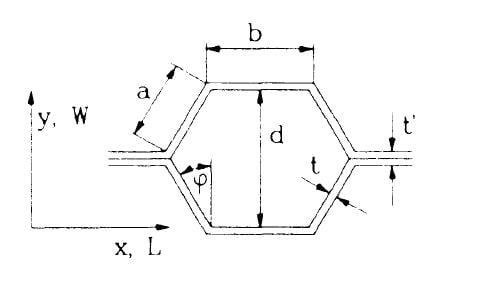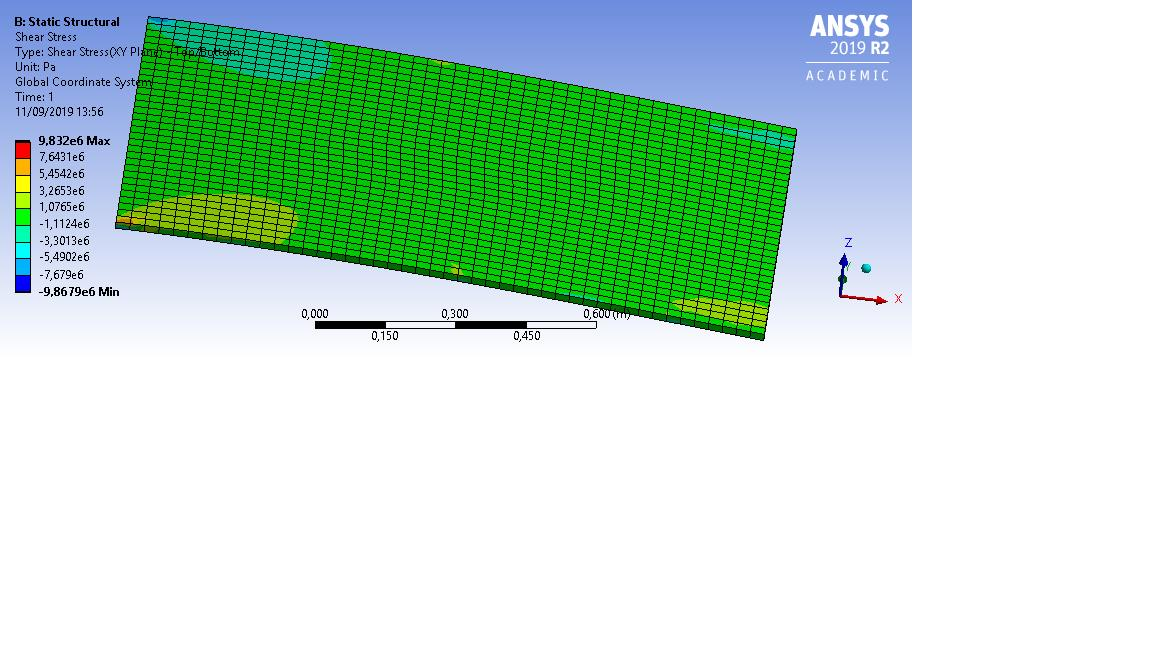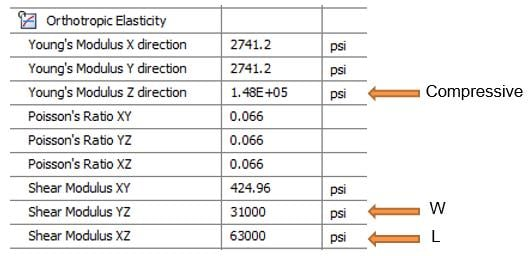## General Mechanical

•Clement08
Subscriber

Dear community,

I'm trying to design a honeycomb core using Ansys. In fact, I'm trying to get the best values for the cell thickness and cell size for a honeycomb made with an isotropic thermoplastic (E1=4GPa, Poisson1=0,38 and density1 = 1400kg/m3, G1=1,38GPa). Sheets are made from a quasi-isotropic composite (E2=60GPa, Poisson2 = 0,3 and density2 = 1800kg/m3) and have a 1mm thickness.

As you can see on the figure below, I’m trying to find the best values for t=t’, a=b and d.After reading this topic (https://forum.ansys.com/forums/topic/honeycomb-structure-adhesive-contact/?order=all#comment-6fd55119-d2d8-453f-bcd7-a80e016d971f) I have decided to model my honeycomb core using a solid orthotropic material.

When one is changing the size of the cell and the thickness of the walls, the equivalent properties of the honeycomb core, governed by the following equations, are going to change :

E*=1,15xE1x(t/l)

Gxz=Gyz=0,58xG1x(t/l)

I have made some assumptions to get this equations : the cell is regular and the properties are the same on the X and Y directions. So, for each cell dimension, I will calculate the equivalent properties and apply it to my core.

Now, I would like to get the maximum shear stress for each case. I'm modelling the sandwich panel using shell elements for the skin and for the core. In order to check if my model is correct, I have fixed the plate at one edge and applied a force on the opposite one. My problem is that my shear result doesn't correspond to my hand calculations. Indeed, for a rectangular section, the maximum shear stress is supposed to be (3/2)x(T/b/h) with T the shear force (here equal to the applied force).

In my case, the section is 960x5mm and the force is equal to 1000N so the maximum shear is supposed to be (3/2)x(1000/5/960)=312kPaThanks for helping me !

•peteroznewman
Subscriber

Dear Clement,

There is a lot to unpack in your post but I immediately reject your assumption "the cell is regular and the properties are the same on the X and Y directions". That is not true.  The properties are very different in the two directions.  Look at the table in the post you linked to where W and L differ by a factor of about 2.If I have other comments, I will make another post.

Kind regards,
Peter

•Clement08
Subscriber

I have made this assumption because I'm not working with common honeycomb core. In general the thickness is not constant so the shear modulus is not the same in the W and L direction. But in my case the cell has a uniform thickness, so I have chosen to use Gibson and Ashby equations because they have used a constant thickness cell.

But I will run some experiments in the future to verify this.

My main problem is about the shear stress calculation. I don't know why my numerical and analytical results are not close to each other.

Many thanks,

Clément

•peteroznewman
Subscriber

Ah, I understand.  The figure above shows a cell with a double thickness wall, but you will have a constant thickness wall.

•Clement08
Subscriber

Yes, sorry about the figure which seems to be more confusing than helpful !